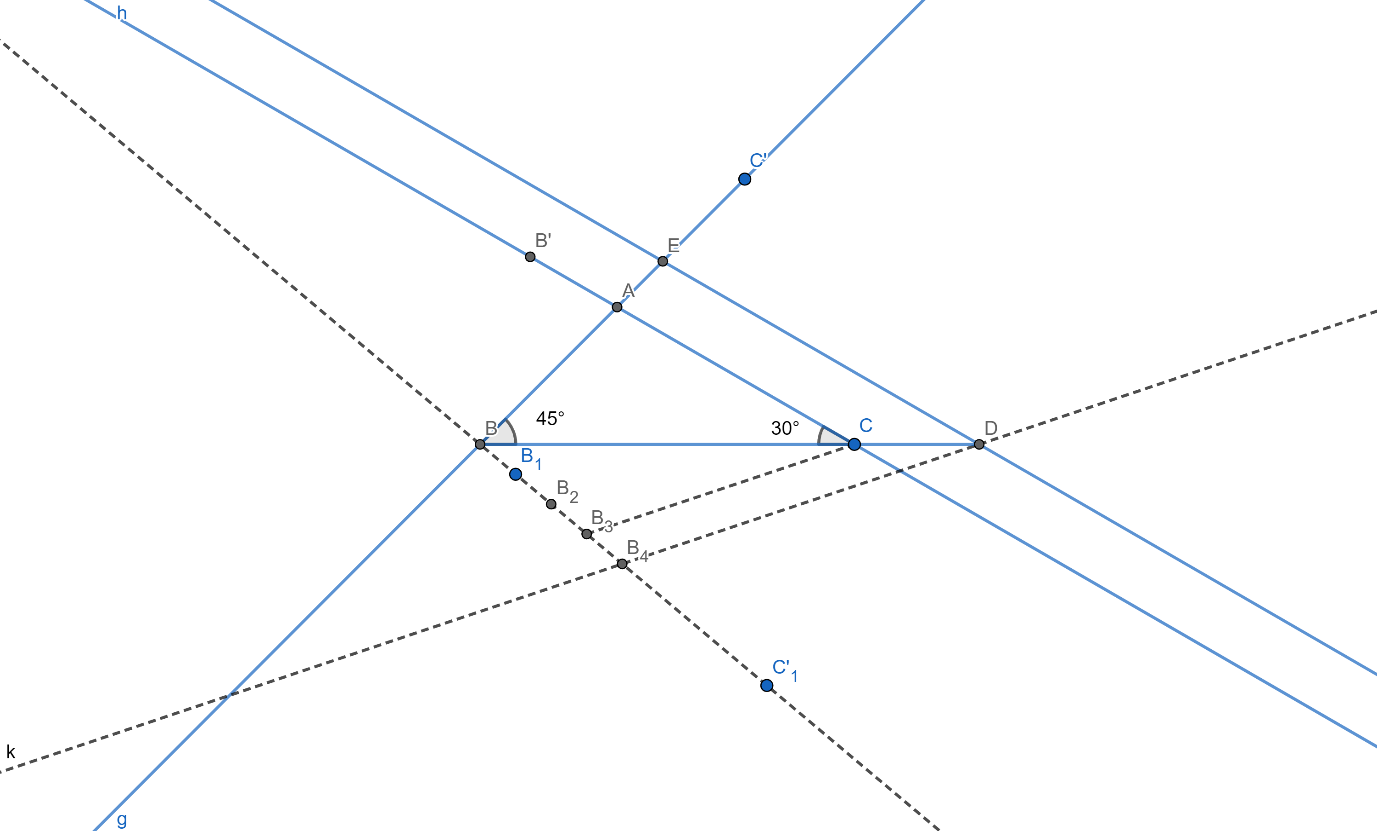QUESTION

# Draw a triangle ABC with BC = 7cm, $\angle \text{B=45}{}^\circ$ and $\angle \text{A=105}{}^\circ$. Then construct another triangle whose sides are $\dfrac{4}{3}$ time the corresponding sides of $\Delta \text{ABC}$. Write the construction.

Draw a triangle ABC with BC = 7cm, $\angle \text{B=45}{}^\circ$ and $\angle \text{A=105}{}^\circ$. Then construct another triangle whose sides are $\dfrac{4}{3}$ time the corresponding sides of $\Delta \text{ABC}$. Write the construction.Calculation of measure of angle C:
We have
\begin{align} & \angle \text{A+}\angle \text{B+}\angle \text{C=180}{}^\circ \\ & \Rightarrow \angle \text{C=180}{}^\circ \text{-150}{}^\circ \text{=30}{}^\circ \\ \end{align}
$Steps$ $for$ $Construction:$
 Draw a line segment BC = 7cm.
 Now Draw angle C’BC $=45{}^\circ$ and B’CB $=30{}^\circ$.
 Let the line C’B and CB’ intersect at A
 ABC is the required triangle.
 Now on the opposite side of BC to A draw and acute angle ${{\text{C}}^{'}}_{1}\text{BC}$.
 Mark four equidistant point ${{\text{B}}_{1}},{{\text{B}}_{2}},{{\text{B}}_{3}}$ and ${{\text{B}}_{4}}$ such that $\text{B}{{\text{B}}_{1}}={{\text{B}}_{1}}{{\text{B}}_{2}}={{\text{B}}_{2}}{{\text{B}}_{3}}={{\text{B}}_{3}}{{\text{B}}_{4}}$
 Join ${{\text{B}}_{3}}$ and C.
 Draw a line parallel to ${{\text{B}}_{3}}\text{C}$ and let it intersect BC produced at D.
 Draw a line parallel to AC through D and let it intersect AC produced at E
 Triangle EBD is the required triangle whose sides are $\dfrac{4}{3}$ times the sides of triangle ABC.

Note: Justification of the construction.
 In triangle ABC, we have BC = 7cm, $\angle \text{B=45}{}^\circ$ and $\angle \text{C=30}{}^\circ$, by construction
Hence $\angle \text{A=180}{}^\circ \text{-}\left( 45{}^\circ +30{}^\circ \right)=105{}^\circ$.
Hence we have created a triangle ABC in which BC = 7cm, $\angle \text{B=45}{}^\circ$ and $\angle \text{A=105}{}^\circ$.
Also, by ASA congruence criterion, the triangle ABC is unique.
 We begin by showing the triangle ABC and EBD are similar.
Since ED||AC, we have
$\angle \text{A=}\angle \text{E}$ and $\angle \text{C=}\angle \text{D}$.
Also, $\angle \text{B}$ is common to the two triangles.
Hence the triangles are similar by AAA similarity. i.e $\Delta \text{ABC}\sim \Delta \text{EBD}$
Now in the triangle $\text{B}{{\text{B}}_{4}}\text{D}$, we have ${{\text{B}}_{3}}\text{C}\parallel {{\text{B}}_{4}}\text{D}$, hence by basic proportionality theorem, we have
$\dfrac{\text{BC}}{\text{BD}}=\dfrac{\text{B}{{\text{B}}_{3}}}{\text{B}{{\text{B}}_{4}}}=\dfrac{3}{4}$
Now since $\Delta \text{ABC}\sim \Delta \text{EBD}$, we have
$\dfrac{\text{AB}}{\text{EB}}=\dfrac{\text{AC}}{\text{ED}}=\dfrac{\text{BC}}{\text{BD}}=\dfrac{3}{4}$
Hence the sides of triangle EBD are $\dfrac{4}{3}$ times the corresponding sides of ABC.
Hence the construction is justified.